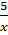### Sample Problem

Solve the nonlinear equation xy + 7x = 5 for y. What is the value of y when x = 5?

#### Solution

xy + 7x = 5

(y + 7) x = 5

y + 7 =y =– 7

When x = 5,

y =– 7

y = -6